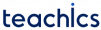0 + 0 = 0
0 + 1 = 1
1 + 0 = 1
1 + 1 = 10

Half adder is a logical circuit that is used to perform this operation. It accepts two binary digits as input and produces two binary digits, a sum and a carry, in its output. The logic symbol of a half adder is given below.

The truth table of a half adder is given below.

A B Cout
0000
0110
1010
1101

The expressions for Sum and Carry can be generated from the following K-Maps.

Observe that the value of Cout is 1 only when both inputs A and B are 1. Therefore Cout can be implemented as AND of two variables.

Cout = AB

Now notice that the value of Sum () is 1 only when inputs A and B are not equal. Therefore the Sum can be expressed as XOR of two variables.

= A⊕B

From these equations, we can implement the logic circuit required for a half adder. The Sum is generated with an XOR gate with two inputs A and B, and the Carry is generated with an AND gate.